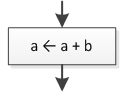Many modern computer languages offers a special set of operators known as compound assignment operators, which can help you write code faster.

## PHP

 Operator Description Example Equivalent to += Addition assignment `\$a += \$b` `\$a = \$a + \$b` -= Subtraction assignment `\$a -= \$b` `\$a = \$a - \$b` *= Multiplication assignment `\$a *= \$b` `\$a = \$a * \$b` /= Division assignment `\$a /= \$b` `\$a = \$a / \$b` %= Modulus assignment `\$a %= \$b` `\$a = \$a % \$b`

## Java, C++, C#

 Operator Description Example Equivalent to += Addition assignment `a += b` `a = a + b` -= Subtraction assignment `a -= b` `a = a - b` *= Multiplication assignment `a *= b` `a = a * b` /= Division assignment `a /= b` `a = a / b` %= Modulus assignment `a %= b` `a = a % b`

## Visual Basic

 Operator Description Example Equivalent to += Addition assignment `a += b` `a = a + b` -= Subtraction assignment `a -= b` `a = a - b` *= Multiplication assignment `a *= b` `a = a * b` /= Division assignment `a /= b` `a = a / b` \= Integer division assignment `a \= b` `a = a \ b` ^= Exponentiation assignment `a ^= b` `a = a ^ b`

## Python

 Operator Description Example Equivalent to += Addition assignment `a += b` `a = a + b` -= Subtraction assignment `a -= b` `a = a - b` *= Multiplication assignment `a *= b` `a = a * b` /= Division assignment `a /= b` `a = a / b` //= Integer division assignment `a //= b` `a = a // b` %= Modulus Assignment `a %= b` `a = a % b` ^= Exponentiation assignment `a ^= b` `a = a ^ b`

Looking at the “Equivalent to” column, it becomes clear that same result can be achieved by just using the classic assignment ( = ) operator. So the question that arises here is why do these operators exist?

The answer is simple: It’s a matter of convenience. Once you start using them, your life finds a different meaning!

Notice: Please keep in mind that flowcharts are a loose method to represent an algorithm. Although the use of compound assignment operators is allowed in flowcharts, this website uses only the commonly accepted operators shown in the “Equivalent to” column. For example, the Java statement` a += b` is represented in a flowchart as